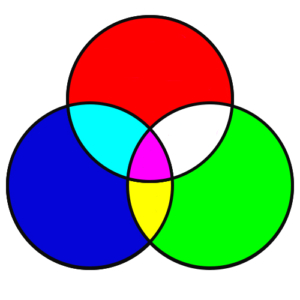# Sets – Definitions of Terms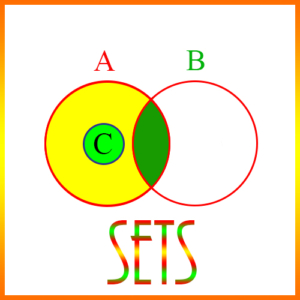Cardinality of a Set: The number of elements in a set.

Difference of Sets: An operation on sets that is similar to subtraction of numbers. If A and B are sets, then their difference, A – B, is a new set, defined as all elements of A that are not in B. Obviously, A – B is not necessarily the same as B – A, since A – B is a subset of A, and B – A is a subset of B.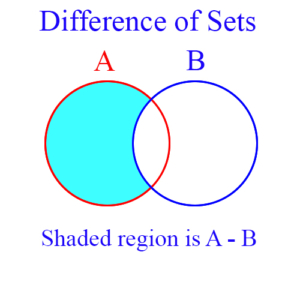Disjoint Sets: Sets which have no common elements are disjoint. This is usually shown by stating their intersection is empty. So, for example, if A and B are disjoint sets, then A ∩ B = ∅. As a specific example, if A = {1, 2} and B = {3, 4, 5}, then A ∩ B = ∅. The figure is a Venn diagram showing that sets A and B don’t overlap or intersect.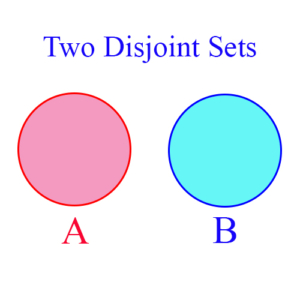Element of a Set: A single member of a set. Examples: If the set A = {1, 2, 3}, then 1, 2, and 3 are its elements. The negative whole numbers are elements of the set of integers. Other elements of the set of integers are 0 and the positive whole numbers.

Empty Set: This a set which has no elements. This may seem counter -intuitive, because sets are collections of objects, but the empty set comes in handy. For example, suppose you take the intersection of two disjoint sets. Since they’re disjoint, their intersection will have no elements in it. But the intersection of any two sets is a new set. So the intersection of two disjoint sets must be a set. It is in fact a set – the empty set. The empty set is usually denoted by the symbol ∅. Example: If two sets, A and B, are disjoint, then A ∩ B = ∅.

Intersection of Sets: The intersection of two sets is itself a new set, and consists of those elements that are in both sets. For example, suppose A = {1, 2, 3} and B = {2, 3, 4}, then the intersection of A and B is {2, 3} – written A ∩ B = {2, 3}. The blue shaded area in the figure represents the intersection of sets A and B.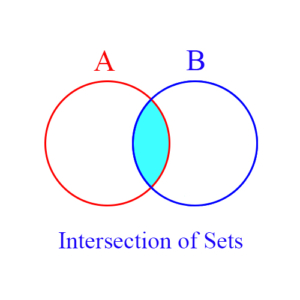Mutually Exclusive Sets: These are sets which are disjoint, as shown in the figure below. That is, they have no elements in common. If A and B are mutually exclusive, then A ∩ B = ∅.Set: A collection of things. In mathematics sets are usually collections of numbers. Examples of sets: All positive integers, all odd integers, all real numbers, {1, 5, -1, 2}. Set operations include set union, intersection, and difference.

Subset: A set whose elements are all elements of another set. Thus, if A = {1, 2, 3} and B = {1, 3}, then B is a subset of A, written B ⊂ A. Other important subsets are: The rational numbers are a subset of the real numbers. The integers are a subset of the rational numbers.

Union of Sets: A set operation which from 2 existing sets creates a new set consisting of all elements that are in either or both of the original sets. Example: If A = {1, 2, 3} and B = {2, 3, 4, 5}, then the set union of A and B, written A ∪ B, is {1, 2, 3, 4, 5}.

Venn Diagram: A pictorial representation of sets, usually with circles or rectangles, which shows the relationships between them, including possibly how they overlap or intersect. The figure below shows 3 sets as circles, and shows their intersections. The maroon portion in the center shows the intersection of all 3 sets.